• 向量坐标相减
2021-04-20 14:36:08

MATLAB部分：

xmap = repmat( linspace( -regionW/2, regionW/2, regionW), regionH, 1 );%linspace [x1,x2,N] 等差数列

ymap = repmat( linspace( -regionH/2, regionH/2, regionH)‘, 1, regionW); %转置

%compute the angle of the vector p1-->p2

vecp1p2 = labelData(2,:) - labelData(1,:);

angle = -atan2(vecp1p2(2), vecp1p2(1)); %角度计算 四象限反正切

widthOfTheRealRegion = norm(vecp1p2) + offset; % 求p1p2点距离，开根号 +offset

midPoint = mean(labelData,1); % 取中点

xmapScaled = xmap * widthOfTheRealRegion / regionW; %对坐标进行scale

ymapScaled = ymap * 24 / regionH;%add for Alexnet

%ymapScaled = ymap * 24 / regionH;%add for Alexnet bywxq

xmapInImage = cos(angle) * xmapScaled + sin(angle) * ymapScaled + midPoint(1); % 顺时针旋转

ymapInImage = -sin(angle) * xmapScaled + cos(angle) * ymapScaled + midPoint(2);

%++++++++++++++++++++++++++++++++++++++

[h, w] = size(xmap);

length = h * w;

xmap = xmap(:);

ymap = ymap(:);

fxmap = floor( xmap ); %向下取整

fymap = floor( ymap );

cxmap = ceil( xmap ); %向上取整

cymap = ceil( ymap );

%插值系数

deltacxx = cxmap - xmap;

deltaxfx = xmap - fxmap;

deltacyy = cymap - ymap;

deltayfy = ymap - fymap;

roi = zeros(length, 1);

C++实现：

//author: fourmi-2018-09-04

#include

#include

#include

#include

#define pi 3.1415926

#define POINT std::pair

#define VECT std::vector<:vector> >

const float regionW=96;

const float regionH=24;

const int offset=24;

float cal_angle(POINT &pt1,POINT &pt2)

{

double angle;

if ((pt2.first-pt1.first)==0)

{

angle=pi/2;

}

else

{

angle=std::abs(atan((pt2.second-pt1.second)/(pt2.first-pt1.first)));

}

return angle;

};

float cal_distance(POINT &pt1,POINT &pt2,const int offset)

{

float distance;

distance=sqrt(pow((pt2.second-pt1.second),2)+pow((pt2.first-pt1.first),2))+offset;

std::cout<

return distance;

}

VECT make_xmap(float regionW,float regionH)

{

VECT array(regionH);

int i,j;

for(i=0;i

array[i].resize(regionW);

for(i=0;i

{

for(j=0;j

{

array[i][j]=-regionW/2+j*(regionW)/(regionW-1);

}

//std::cout<

}

return array;

}

VECT make_ymap(float regionW,float regionH)

{

VECT array(regionH);

int i,j;

for(i=0;i

array[i].resize(regionW);

for(i=0;i

{

for(j=0;j

{

array[j][i]=-regionH/2+j*(regionH)/(regionH-1);

//std::cout<

}

//std::cout<<:endl>

}

return array;

}

VECT Scaled(VECT array,float scale)

{

VECT result(array.size());

int i,j;

for(i=0;i

result[i].resize(array.size());

for(i=0;i

{

for(j=0;j

{

result[i][j]=array[i][j]*scale;

//std::cout<

}

//std::cout<<:endl>

}

return result;

}

VECT ADD(VECT array0,VECT array1)

{

VECT result(array0.size());

int i,j;

for(i=0;i

result[i].resize(array0.size());

for(i=0;i

{

for(j=0;j

{

result[i][j]=array0[i][j]+array1[i][j];

}

}

return result;

}

VECT ADD_const(VECT array0,float num)

{

VECT result(array0.size());

int i,j;

for(i=0;i

result[i].resize(array0.size());

for(i=0;i

{

for(j=0;j

{

result[i][j]=array0[i][j]+num;

}

}

return result;

}

std::vector round(std::vector array,char kind)

{

std::vector result(array.size());

int n=0;

for(int i=0;i

{

if (kind==‘f‘)

{

result[i]=floor(array[i]);

}

else

{

result[i]=ceil(array[i]);

}

}

return result;

}

std::vector sub_vector(std::vector array0,std::vector array1)

{

std::vector result(array0.size());

int n=0;

for(int i=0;i

{

result[i]=array0[i]-array1[i];

}

return result;

}

std::vector change_format(VECT array)

{

std::vector result(array.size()*array.size());

int n=0;

for(int i=0;i

{

for(int j=0;j

{

result[n]=array[j][i];

n++;

}

}

return result;

}

VECT zeros(int length,float num)

{

VECT result(length);

int i,j;

for(i=0;i

result[i].resize(num);

for(i=0;i

{

for(j=0;j

{

result[i][j]=0;

}

}

return result;

}

POINT * compare_2_points(POINT &pt1,POINT &pt2,double angle,int offset)

{

int x_left,x_right,y_down,y_up;

POINT new_pt1,new_pt2;

static POINT arr;

/*

pt1.first=std::abs(pt1.first);

pt2.first=std::abs(pt2.first);

pt1.second=-std::abs(pt1.second);

pt2.second=-std::abs(pt2.second);

*/

if (pt2.first

{

x_left=pt2.first;

x_right=pt1.first;

}

else

{

x_left=pt1.first;

x_right=pt2.first;

}

std::cout<

if(pt2.second

{

y_down=pt2.second;

y_up=pt1.second;

}

else

{

y_down=pt1.second;

y_up=pt2.second;

}

std::cout<

new_pt1.first=x_left;

new_pt1.second=y_up;

new_pt2.first=x_right;

new_pt2.second=y_down;

arr=new_pt1;

arr=new_pt2;

return arr;

};

POINT make_mid(POINT &pt1,POINT &pt2)

{

POINT midPoint;

midPoint.first=(pt1.first+pt2.first)/2;

midPoint.second=(pt1.second+pt2.second)/2;

return midPoint;

}

POINT * make_new_points(POINT &pt1,POINT &pt2,double angle,int offset)

{

double x_offset,y_offset;

POINT new_pt1,new_pt2;

static POINT arr;

x_offset=(offset)/2.0*cos(angle);

y_offset=(offset)/2.0*sin(angle);

new_pt1.first=pt1.first-x_offset;

new_pt1.second=pt1.second+y_offset;

new_pt2.first=pt2.first+x_offset;

new_pt2.second=pt2.second-y_offset;

arr=new_pt1;

arr=new_pt2;

return arr;

};

int main()

{

double angle;

VECT xmap,ymap,xmapScaled,ymapScaled,xmapInImage,ymapInImage;

POINT point1(171,213);

POINT point2(171,145);

POINT new_pt1,new_pt2,midPoint;

POINT * arr;

float widthOfTheRealRegion ;

int h,w,length;

std::vector xmapInImage0,ymapInImage0,fxmap,fymap,cxmap,cymap,deltacxx,deltaxfx,deltacyy,deltayfy,roi;

xmap=make_xmap(regionW,regionH);

ymap=make_ymap(regionW,regionH);

arr=compare_2_points(point1,point2,0,0);

new_pt1=arr;

new_pt2=arr;

angle=cal_angle(new_pt1,new_pt2);

std::cout<

widthOfTheRealRegion=cal_distance(new_pt1,new_pt2,offset);

midPoint=make_mid(new_pt1,new_pt2);

std::cout<

xmapScaled=Scaled(xmap,widthOfTheRealRegion/regionW);

ymapScaled=Scaled(ymap,24/regionH);

h=xmapInImage.size();

w=xmapInImage.size();

length=h*w;

xmapInImage0=change_format(xmapInImage);

ymapInImage0=change_format(ymapInImage);

fxmap=round(xmapInImage0,‘f‘);

fymap=round(ymapInImage0,‘f‘);

cxmap=round(xmapInImage0,‘c‘);

cymap=round(ymapInImage0,‘c‘);

deltacxx=sub_vector(cxmap,xmapInImage0);

deltaxfx=sub_vector(xmapInImage0,fxmap);

deltacyy=sub_vector(cymap,ymapInImage0);

deltayfy=sub_vector(ymapInImage0,fymap);

roi=change_format(zeros(length,1));

for (int i=0;i

{

std::cout<

}

//std::cout<

//std::cout<

/*

arr=make_new_points(new_pt1,new_pt2,angle,offset);

new_pt1=arr;

new_pt2=arr;

std::cout<

std::cout<

for(int i=0;i

{

for(int j=0;j

{

std::cout<

}

std::cout<<:endl>

std::cout<

}

*/

return 0;

}

原文：https://www.cnblogs.com/fourmi/p/9585978.html

更多相关内容
• 减法：等于各分量相减 ...在Unity中 两个向量相减 后的向量的起始坐标和a，b的起点相同（如下图） 使用 ti-t2后得到的向量是这样的（如下图） 应用：计算两点之间的距离和相对方向 代码表示： ...

减法：等于各分量相减

公式：[x1,y1,z1]-[x2,y2,z2]=[x1-x2,y1-y2,z1-z2]

几何意义：向量a，向量b相减，理解为以b的终点为始点，以a的终点为终点的向量，方向由b指向a (指向被减数)

在Unity中 两个向量相减 后的向量的起始坐标和a，b的起点相同（如下图）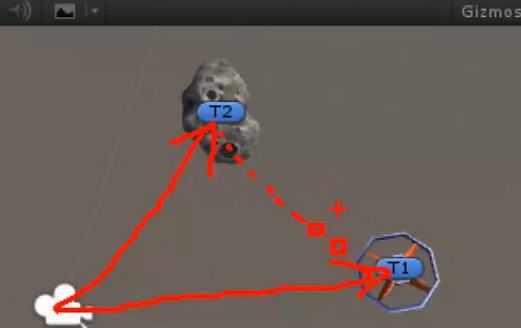使用 ti-t2后得到的向量是这样的（如下图）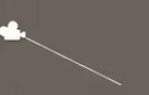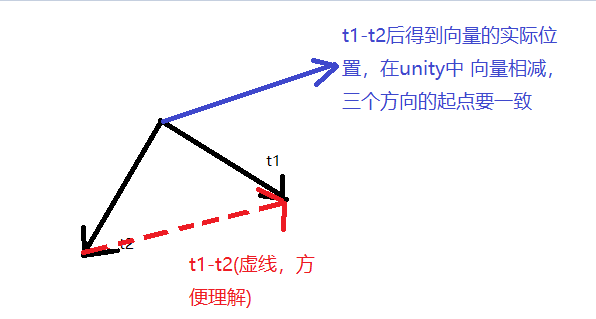应用：计算两点之间的距离和相对方向

代码表示：


//相减
void Demo03()
{
Vector3 dir = t1.position - t2.position;
//方向：指向被减向量（t1）
//大小：两点间距
//注意：实际位置要平移到坐标原点（这里 t1，t2都是世界坐标）
//t3沿着dir方向移动 dir.normalized:获取方向，不受大小的影响
t3.Translate(dir.normalized);
Debug.DrawLine(Vector3.zero,dir);
}


实际情况：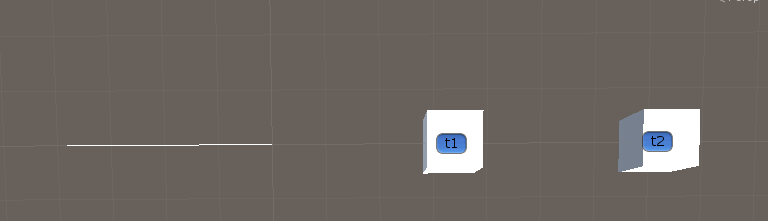展开全文• av28779788?p=?视频学习笔记 当前为-->第四部分：3D数学 向量 ...向量的模 ...求模,在unity中随便添加1个物体,再将下例脚本拖拽其上,运行结果如图: ...如上,白线为模长--注:为方便,本章代码中摄像机均位于...向量相减...

av28779788?p=?视频学习笔记

• 当前为-->第四部分：3D数学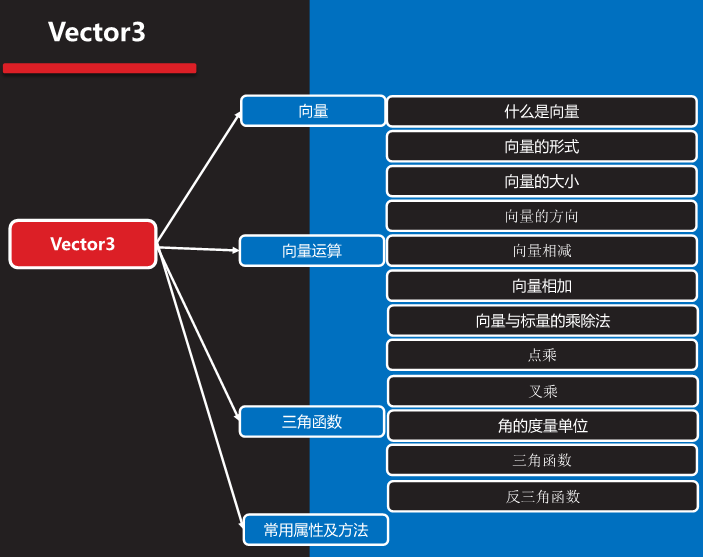# 向量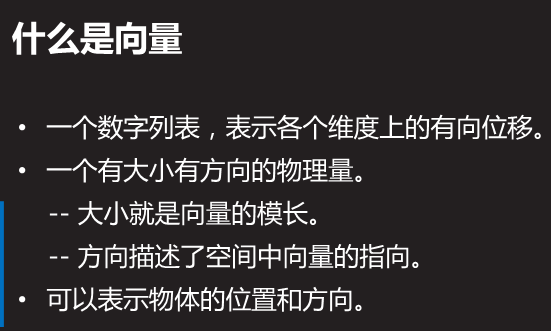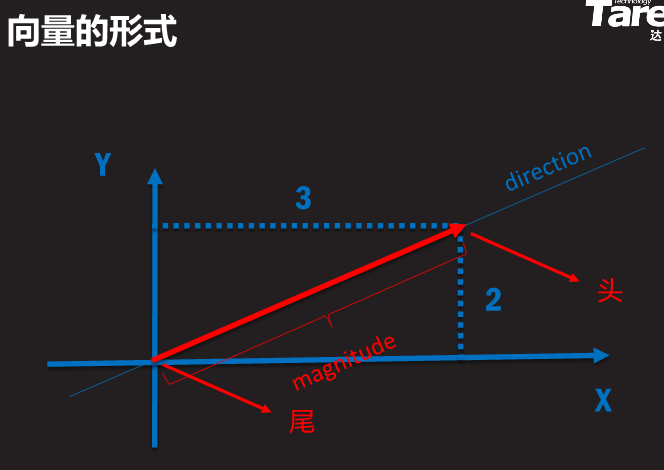## 向量的模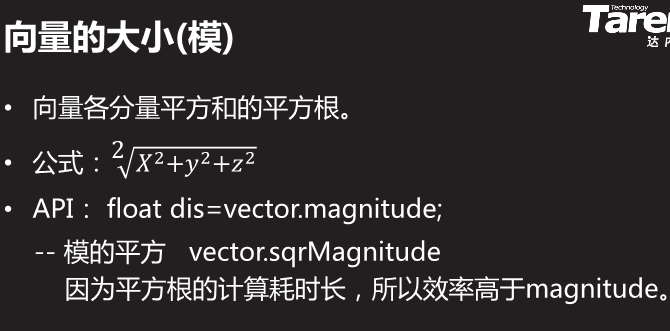求模,在unity中随便添加1个物体,再将下例脚本拖拽其上,运行结果如图: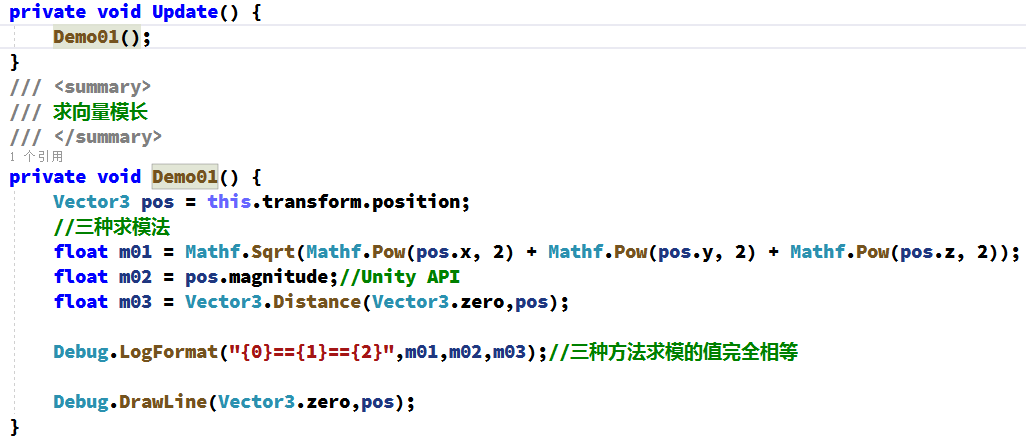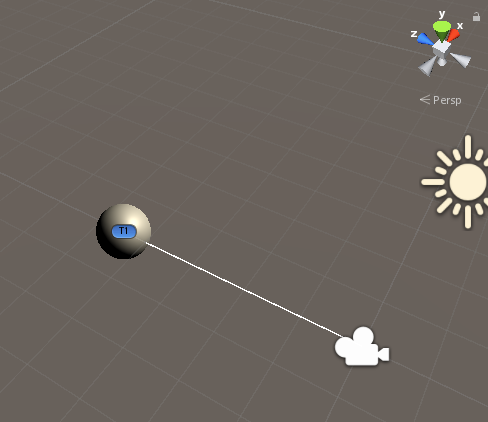如上,白线为模长--注:为方便,本章代码中摄像机均位于世界坐标原点(0,0,0)

## 向量的方向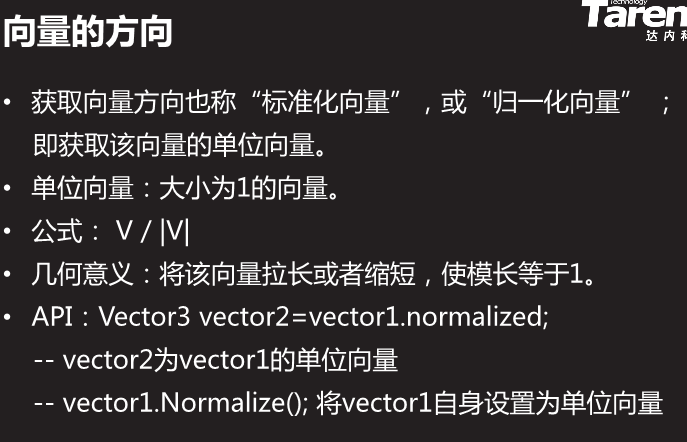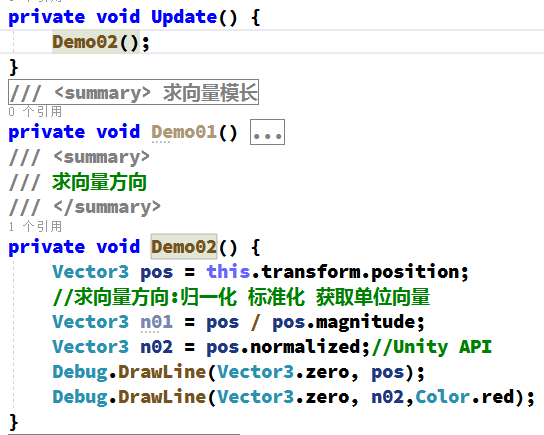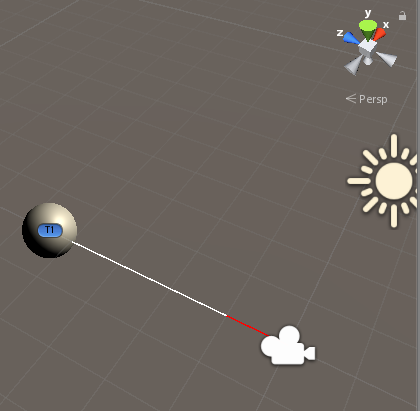如上,红线为单位向量-向量方向-归一化向量.

# 向量运算(1)## 向量相减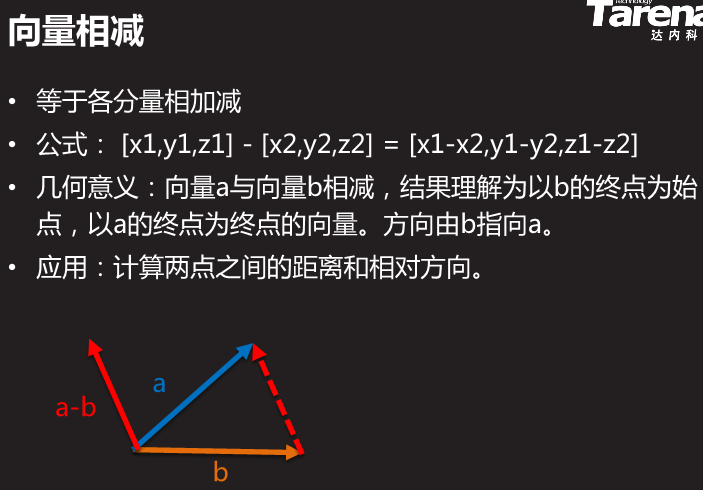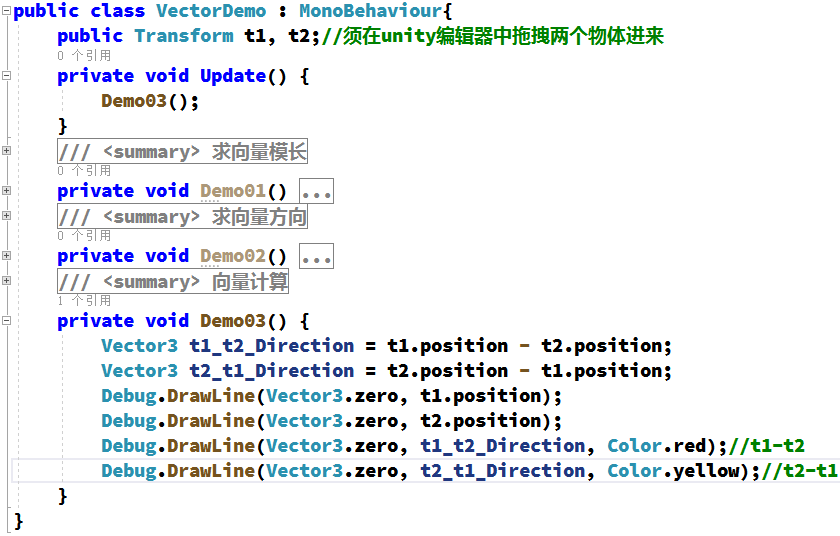为了便于观察理解,以下2个物体Y坐标皆是0,运行后感觉结果怪怪的,改成ISO正交模式后观察就容易理解了: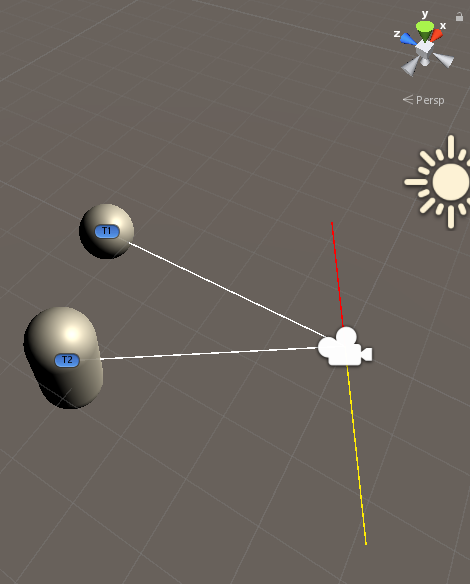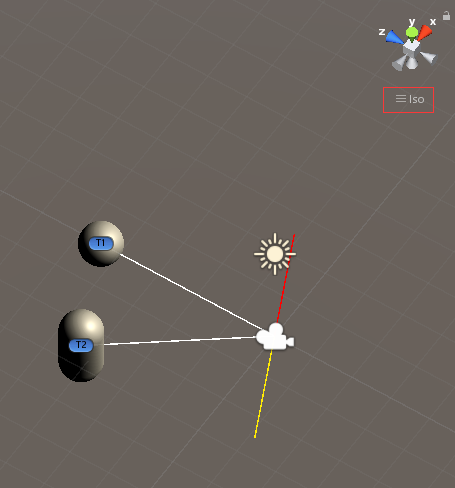如上,红线为T1-T2;黄线为T2-T1.以下为草图辅助理解.虚线为我们理解的T2-T1但实际并不存在.因为所有向量起点必须是世界坐标原点0,0,0.

.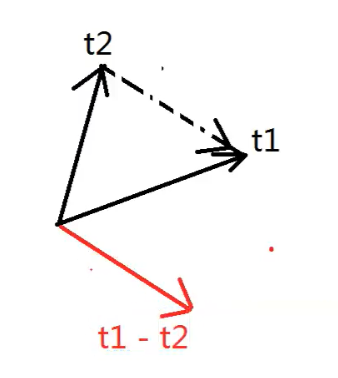修改1下代码,再拖放1个物体T3到场景中,将T3和T2放一起.然后t3也挂在脚本上.下例代码按空格键t3(相当于子弹)会向t1移动: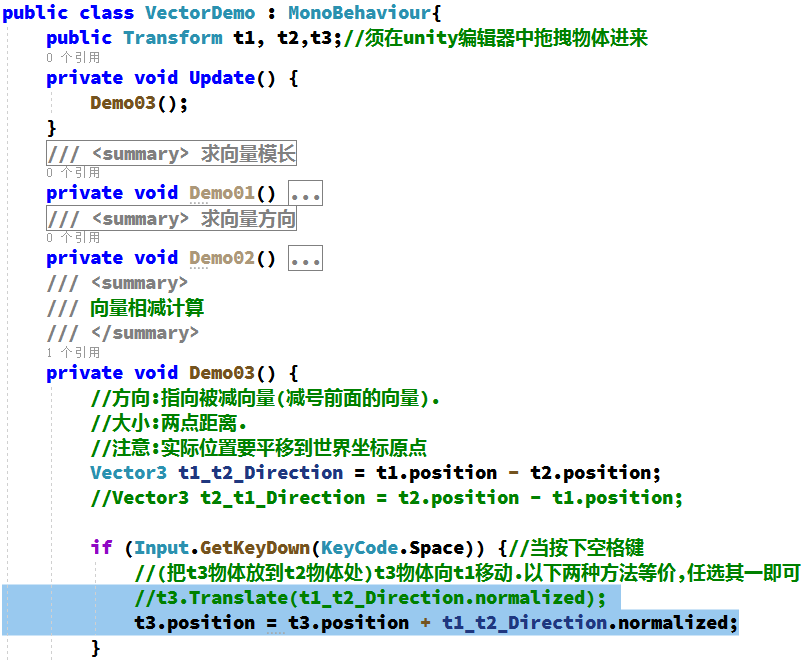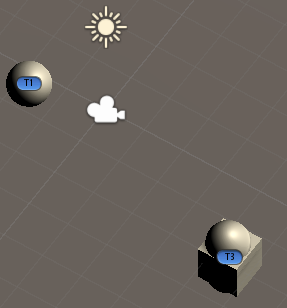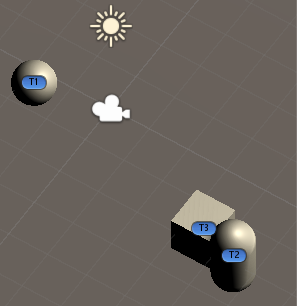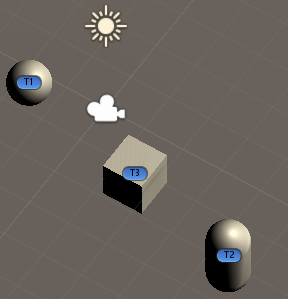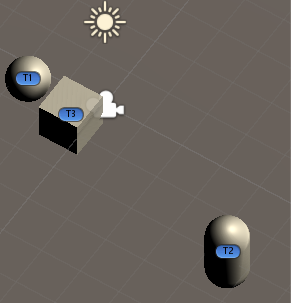## 向量相加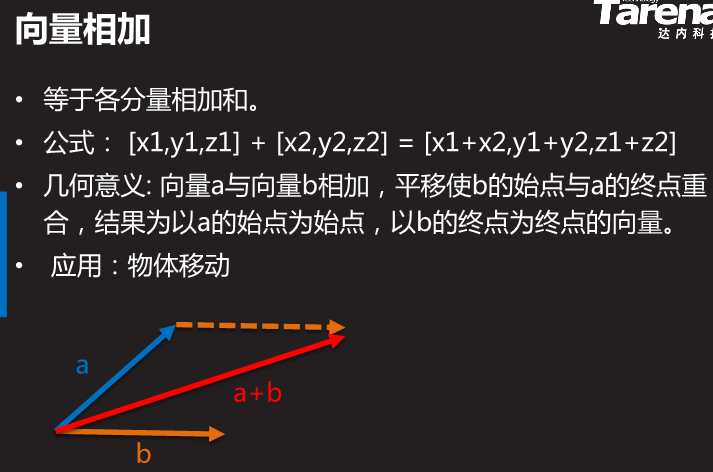## 向量乘除标量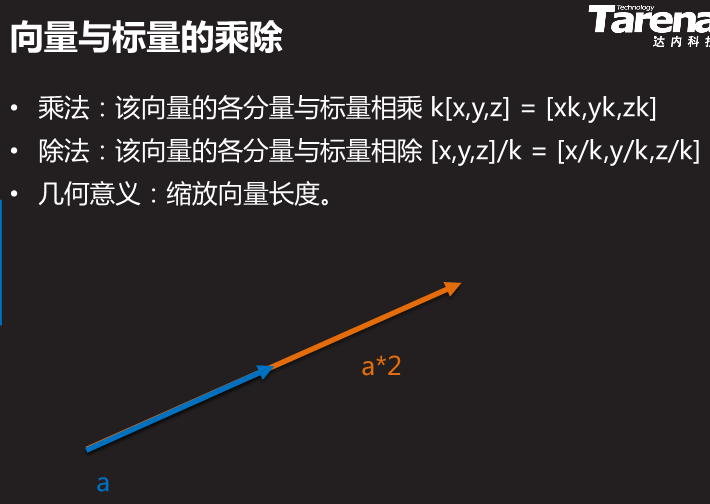# 三角函数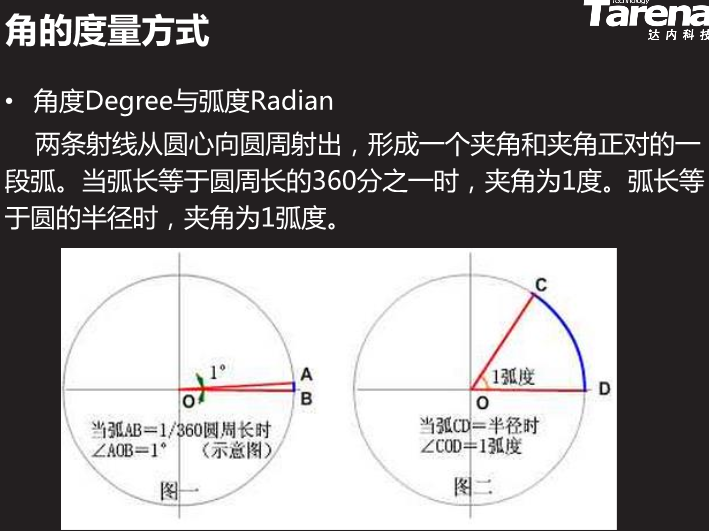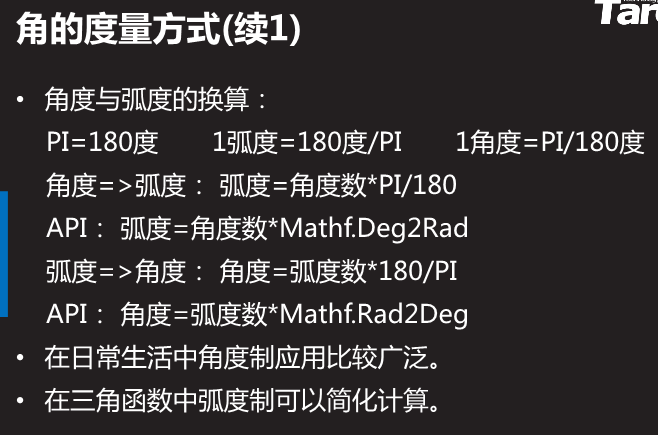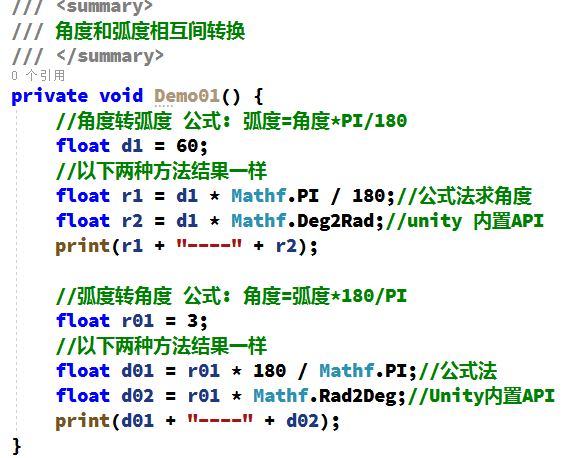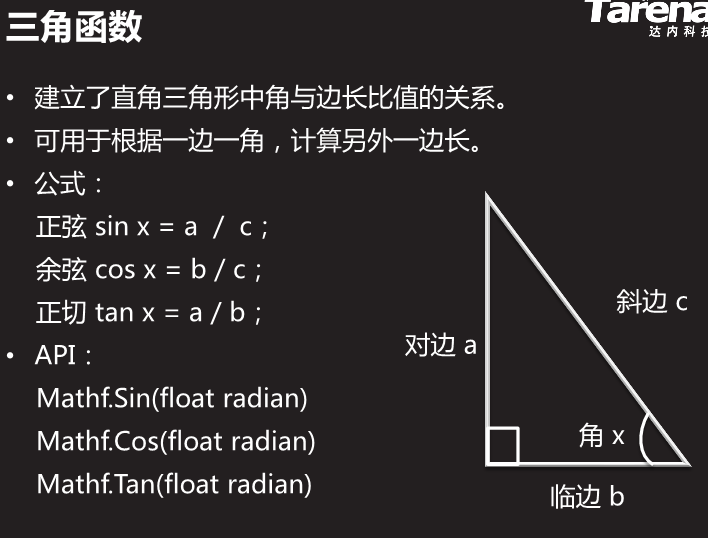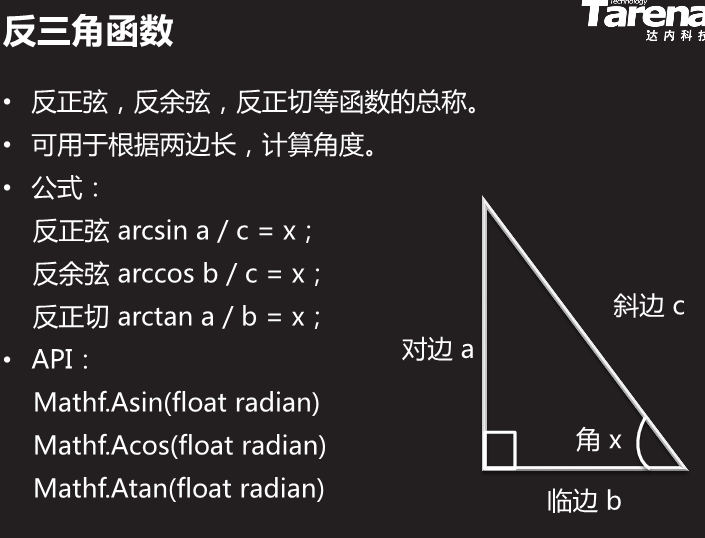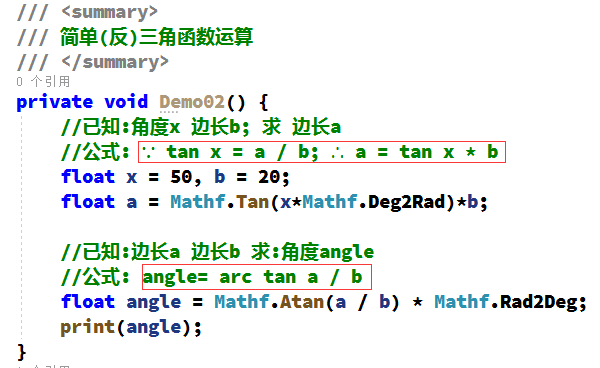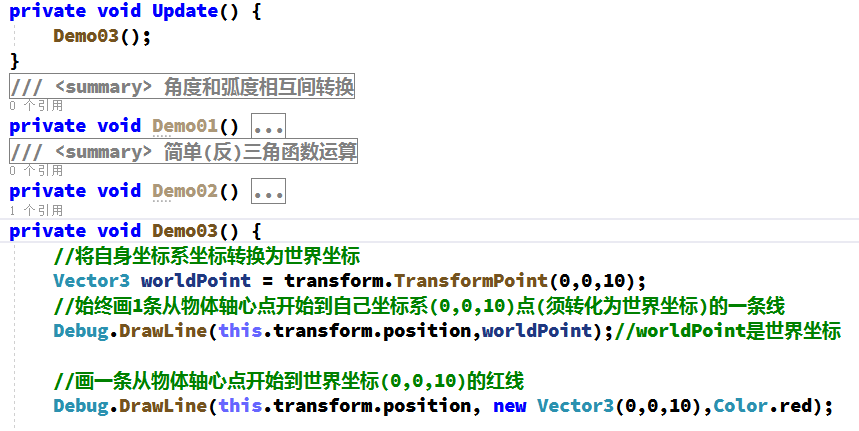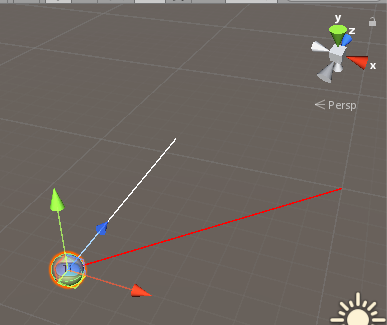如上,白线会随物体运动而变化(永远指向物体自身坐标的(0,0,10)).
不管物体如何运动,红线的终点始终在世界坐标(0,0,10).
-------------------------------------------------------------------

练习:计算物体右前方30度,10米远的坐标(如图是横X轴,竖Z轴,Y轴忽略不计)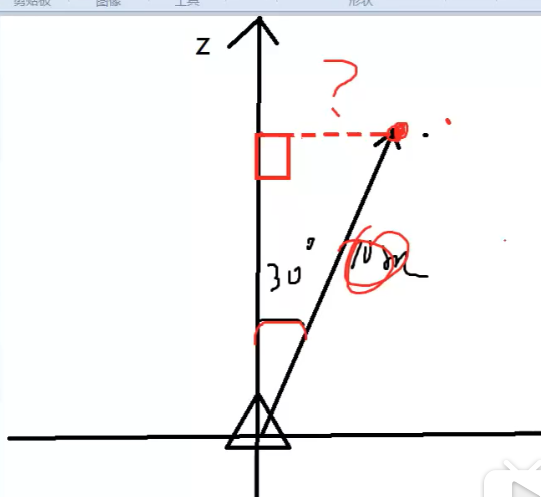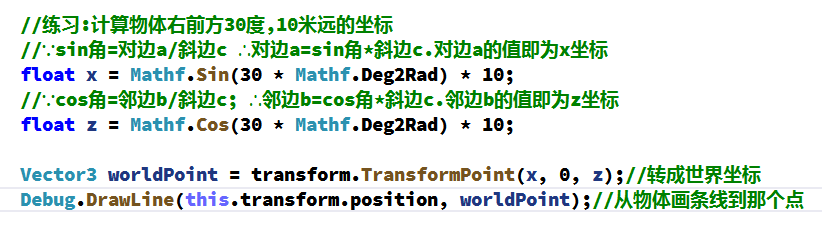如下,白线终点即为所求坐标点.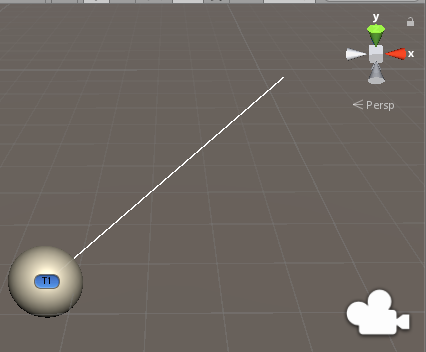# 向量运算(2)## 点乘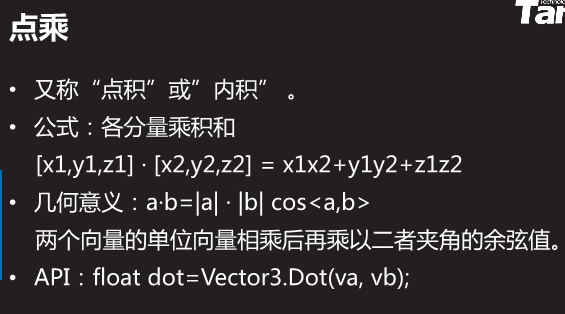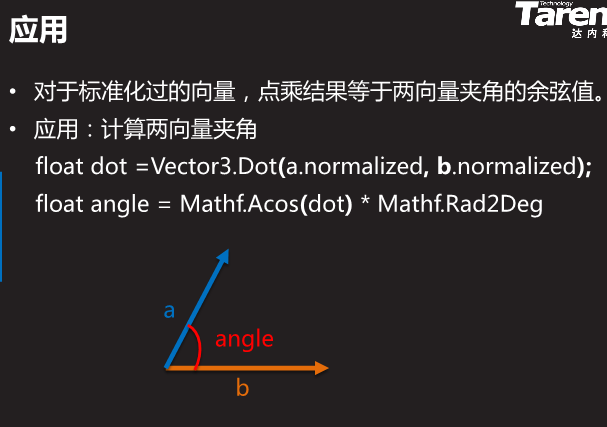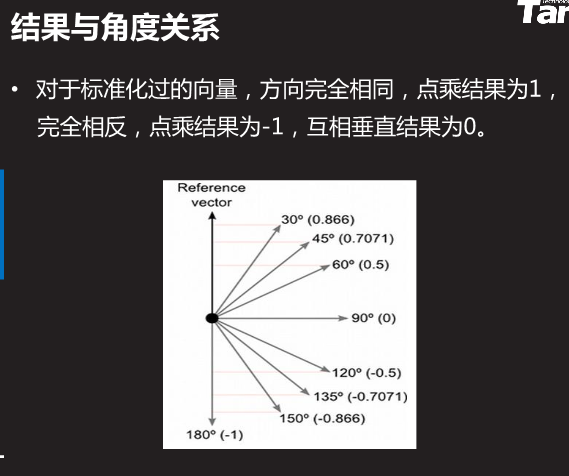注意:根据点乘结果计算夹角范围为0-180度.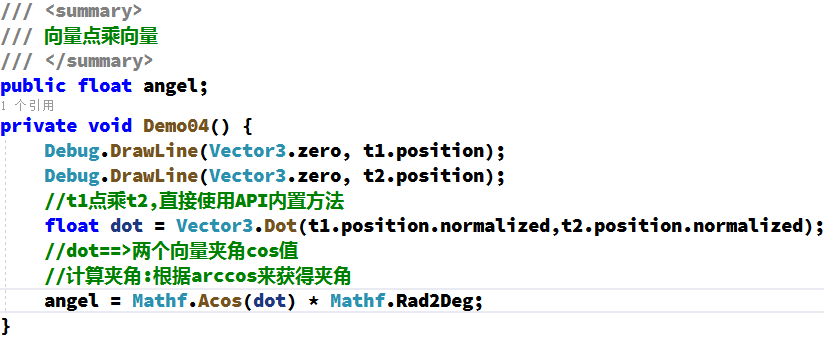结果如下图: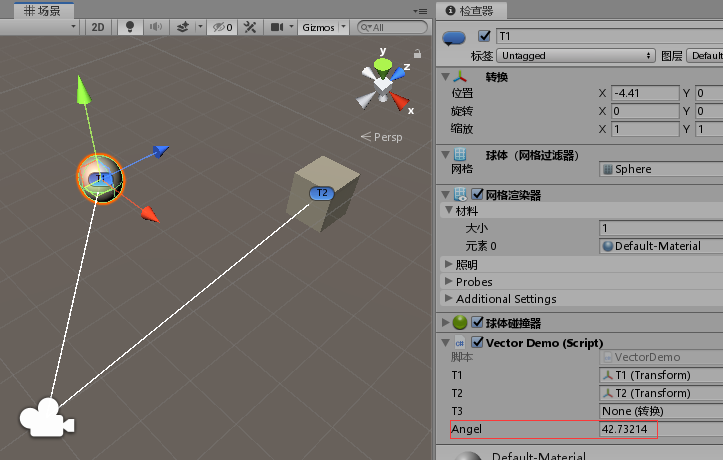假如我们在代码中添加判断夹角是否大于60度的逻辑,以下两种写法都可以.其中第二种方法可以避免做一次arccos运算.

当然,也可以用查表法.即在程序开始之时把各个角度的正弦余弦等提前算好存入数组中.这样在游戏中需要时不必计算(因为计算通常都是在Update中频繁运算,比较浪费计算机资源)直接读取数组相应元素即可.相当于把正弦表余弦表存入计算机,需要时读取: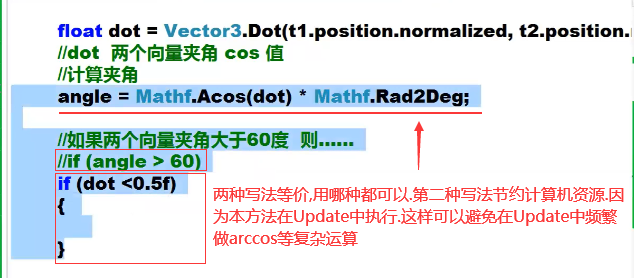## 叉乘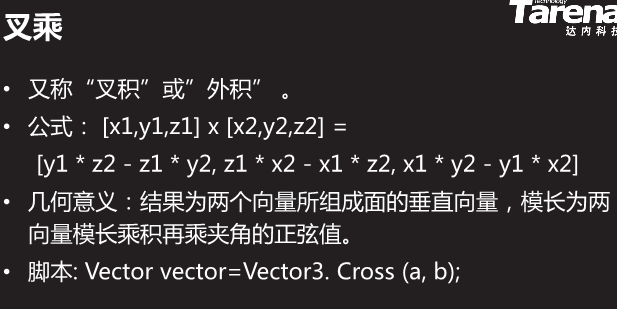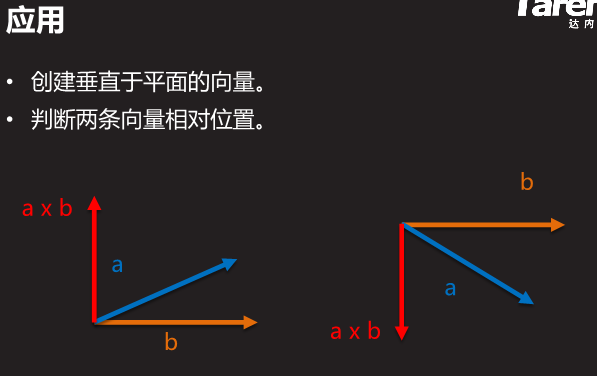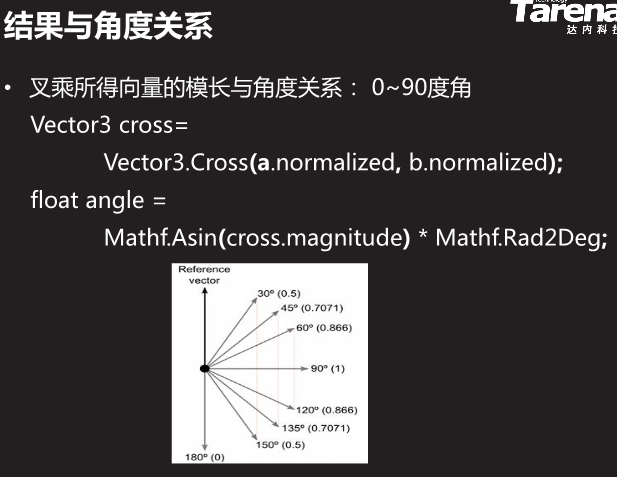结合点乘与叉乘获取两向量夹角,取值范围0-360度: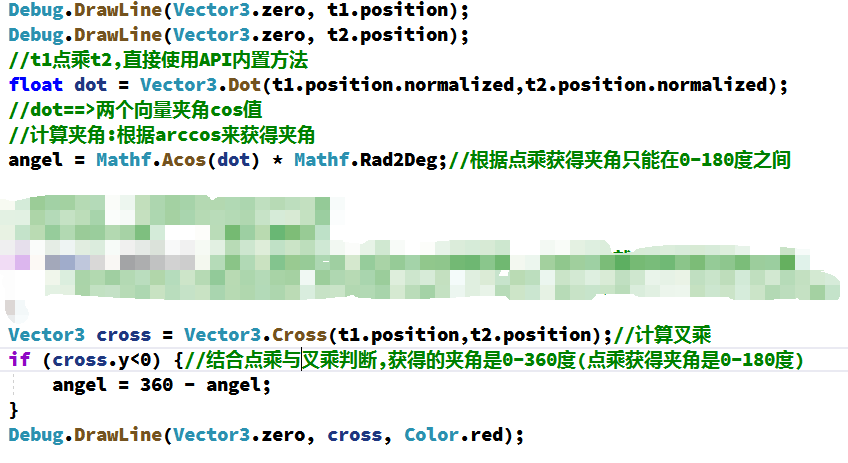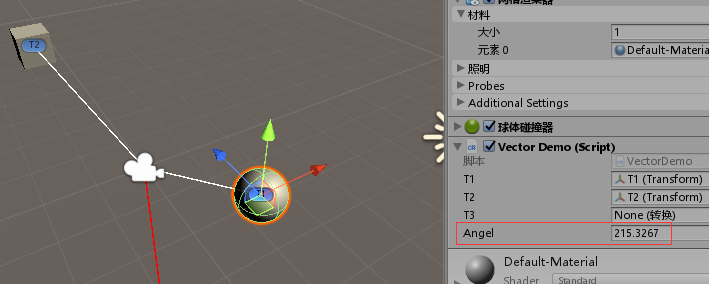注意:结合点乘与叉乘,可判断夹角在0-360度(单独用叉乘获得的夹角在0-90度,一般不用)

练习:敌人对玩家发动攻击的逻辑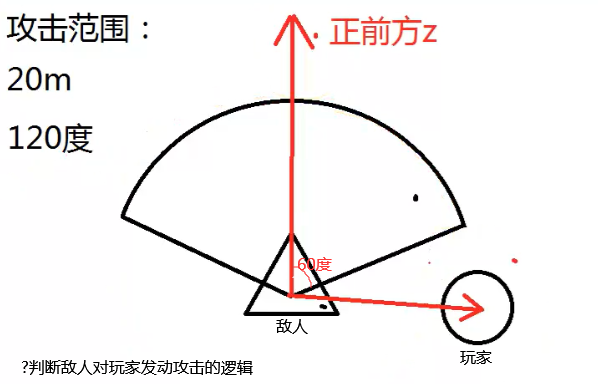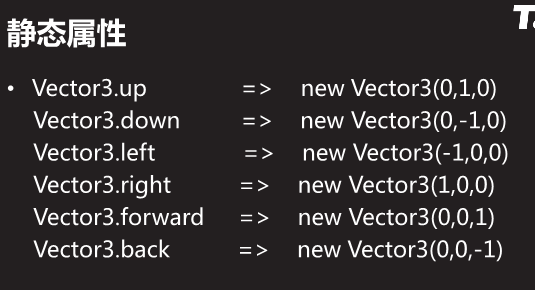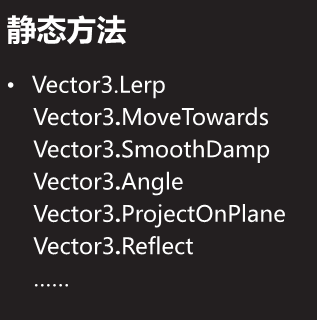展开全文• 设a=(x,y),b=(x',y').1、向量的加法向量的加法满足平行四边形法则和三角形法则.AB+BC=AC.a+b=(x+x',y+y').a+0=0+a=a.向量加法的运算律：交换律：a+b=b+a；结合律：(a+b)+c=a+(b+c).2、向量的减法如果a、b是互为相反...

设a=(x,y),b=(x',y').

1、向量的加法

向量的加法满足平行四边形法则和三角形法则.

AB+BC=AC.

a+b=(x+x',y+y').

a+0=0+a=a.

向量加法的运算律：

交换律：a+b=b+a；

结合律：(a+b)+c=a+(b+c).

2、向量的减法

如果a、b是互为相反的向量,那么a=-b,b=-a,a+b=0.0的反向量为0

AB-AC=CB.即“共同起点,指向被减”

a=(x,y) b=(x',y') 则 a-b=(x-x',y-y').

4、数乘向量

实数λ和向量a的乘积是一个向量,记作λa,且∣λa∣=∣λ∣•∣a∣.

当λ＞0时,λa与a同方向；

当λ＜0时,λa与a反方向；

当λ=0时,λa=0,方向任意.

当a=0时,对于任意实数λ,都有λa=0.

注：按定义知,如果λa=0,那么λ=0或a=0.

实数λ叫做向量a的系数,乘数向量λa的几何意义就是将表示向量a的有向线段伸长或压缩.

当∣λ∣＞1时,表示向量a的有向线段在原方向(λ＞0)或反方向(λ＜0)上伸长为原来的∣λ∣倍；

当∣λ∣＜1时,表示向量a的有向线段在原方向(λ＞0)或反方向(λ＜0)上缩短为原来的∣λ∣倍.

数与向量的乘法满足下面的运算律

结合律：(λa)•b=λ(a•b)=(a•λb).

向量对于数的分配律(第一分配律)：(λ+μ)a=λa+μa.

数对于向量的分配律(第二分配律)：λ(a+b)=λa+λb.

数乘向量的消去律：① 如果实数λ≠0且λa=λb,那么a=b.② 如果a≠0且λa=μa,那么λ=μ.

3、向量的的数量积

定义：已知两个非零向量a,b.作OA=a,OB=b,则角AOB称作向量a和向量b的夹角,记作〈a,b〉并规定0≤〈a,b〉≤π

定义：两个向量的数量积(内积、点积)是一个数量,记作a•b.若a、b不共线,则a•b=|a|•|b|•cos〈a,b〉；若a、b共线,则a•b=+-∣a∣∣b∣.

向量的数量积的坐标表示：a•b=x•x'+y•y'.

向量的数量积的运算律

a•b=b•a(交换律)；

(λa)•b=λ(a•b)(关于数乘法的结合律)；

(a+b)•c=a•c+b•c(分配律)；

向量的数量积的性质

a•a=|a|的平方.

a⊥b 〈=〉a•b=0.

|a•b|≤|a|•|b|.

向量的数量积与实数运算的主要不同点

1、向量的数量积不满足结合律,即：(a•b)•c≠a•(b•c)；例如：(a•b)^2≠a^2•b^2.

2、向量的数量积不满足消去律,即：由 a•b=a•c (a≠0),推不出 b=c.

3、|a•b|≠|a|•|b|

4、由 |a|=|b| ,推不出 a=b或a=-b.

4、向量的向量积

定义：两个向量a和b的向量积(外积、叉积)是一个向量,记作a×b.若a、b不共线,则a×b的模是：∣a×b∣=|a|•|b|•sin〈a,b〉；a×b的方向是：垂直于a和b,且a、b和a×b按这个次序构成右手系.若a、b共线,则a×b=0.

向量的向量积性质：

∣a×b∣是以a和b为边的平行四边形面积.

a×a=0.

a‖b〈=〉a×b=0.

向量的向量积运算律

a×b=-b×a；

(λa)×b=λ(a×b)=a×(λb)；

(a+b)×c=a×c+b×c.

注：向量没有除法,“向量AB/向量CD”是没有意义的.

向量的三角形不等式

1、∣∣a∣-∣b∣∣≤∣a+b∣≤∣a∣+∣b∣；

① 当且仅当a、b反向时,左边取等号；

② 当且仅当a、b同向时,右边取等号.

2、∣∣a∣-∣b∣∣≤∣a-b∣≤∣a∣+∣b∣.

① 当且仅当a、b同向时,左边取等号；

② 当且仅当a、b反向时,右边取等号.

定比分点

定比分点公式(向量P1P=λ•向量PP2)

设P1、P2是直线上的两点,P是l上不同于P1、P2的任意一点.则存在一个实数 λ,使 向量P1P=λ•向量PP2,λ叫做点P分有向线段P1P2所成的比.

若P1(x1,y1),P2(x2,y2),P(x,y),则有

OP=(OP1+λOP2)(1+λ)；(定比分点向量公式)

x=(x1+λx2)/(1+λ),

y=(y1+λy2)/(1+λ).(定比分点坐标公式)

我们把上面的式子叫做有向线段P1P2的定比分点公式

三点共线定理

若OC=λOA +μOB ,且λ+μ=1 ,则A、B、C三点共线

三角形重心判断式

在△ABC中,若GA +GB +GC=O,则G为△ABC的重心

[编辑本段]向量共线的重要条件

若b≠0,则a//b的重要条件是存在唯一实数λ,使a=λb.

a//b的重要条件是 xy'-x'y=0.

零向量0平行于任何向量.

[编辑本段]向量垂直的充要条件

a⊥b的充要条件是 a•b=0.

a⊥b的充要条件是 xx'+yy'=0.

零向量0垂直于任何向量.不知你要的是不是这些?

解析看不懂？求助智能家教解答查看解答

更多答案(2)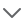展开全文• 模：向量的长度=>sqr(x^2+y^2+z^2) //各向量的平方相加，在开方 Unity API: Vector.magnitude 单位向量：长度为1的向量叫单位向量(1,1,1) //各个分量分别除以magnitude模长就等于单位向量 公式: Vector v1= ...unity unity3d
• 实现三维向量之间的加法与减法的功能。 ####相关知识 在 Python 面向对象编程中，类的特殊方法在形式上是前后各有 2 个下划线，作用是实现与某个运算符或内置函数的对应关系。例如，如果某个类实现了__len__()这个...python
• 注：两个向量相减，则表示两个向量起点的字母必须相同；差向量的终点指向被减向量的终点。平行四边形定则解决向量加法的办法将两个向量平移至公共起点，以向量的两条边作平行四边形，结果为公共起点的对角线。平...
• 终点坐标减去起点坐标（减数指向被减数） 3.点乘，点积，标量积，内积 （1）定义 每对分量乘积之和：a·b=axbx+ayby+azbz 两模相乘再乘于夹角余弦：a·b=|a||b|cosθ （2）矢量投影 u为单位向量 a·u为a...unity
• 向量（又称适量）是游戏开发中非常... （1）加减法：向量的加法（减法）为各个分量的相加（相减）。 a(2,2,3)+b(1,1,1) = c(2+1,2+1,3+1); a(2,2,3)-b(1,1,1) = c(2-1,2-1,3-1); 作用：改变物体的坐标。 ...unity
• ## 向量

2019-11-06 18:02:11
一个向量的坐标等于表示此向量的有向线段的终点坐标减去始点的坐标 ...向量减法 (数学上，两个向量坐标相减） OA-OB=BA 共起点，指被减向量（减向量[OB]终点 指向 被减向量[OA]终点） OA-OB=OA+(-OB)=OA...
• 一、向量的加法两个向量做加法运算就是向量的加法，是一种向量的运算。首先我们来看图像。向量加法图像向量的加法口诀：首尾相连，首连尾，方向指向末向量。以第一个向量的起点为起点，以第二个向量的终点为终点的...
• 向量复习（一）：定义、求解、四则运算、点积和叉积
•unity
• ## Cocoscreator 向量计算

千次阅读 2020-05-08 13:59:29
向量相减，得到目标向量 起点A(200,100) 目标B(100,200) A到B的向量 = B点 - A点。 那我们只需要将目标对象的位置 - 自己的位置，即可得到方向向量,方向向量转换为角度 需要认清一个隐含变量，就是这个角度的基准是...
• unity3D数学基础坐标系左右沙鸥坐标系功能快捷键合理的创建标题，有助于目录的生成如何改变文本的样式插入链接与图片如何插入一段漂亮的代码片生成一个适合你的列表创建一个表格设定内容居中、居左、居右SmartyPants...unity
• 如何用matlab函数求两个区域相减，如下图所示，我想求用外面的大图减去里面的小图组成大图的向量为：x =[9.9811 10.0441 9.9181 10.1071 10.0441 10.1071 9.9181 9.9811 9.9811...10.1071 10.0441 10.1071 11.1146 ...
• ## MATLAB曲线相减

千次阅读 2021-05-12 20:42:01
现有两条曲线(x⃗1\vec x1x1,y⃗1\vec y1y​1),(x⃗2\vec x2x2,y⃗2\vec y2y​2)，两条曲线的坐标不一定对齐，点数不一定相同，即 x⃗1≠x⃗2\vec x1\neq \vec x2x1​=x2，现要求两条曲线的差值。 解决方法 在网上...matlab
• Vector arithmetic is fundamental to 3D graphics, physics and animation and it is useful to understand it in depth to get the most out of Unity. Below are descriptions of the main operations and some ...
• 一、坐标系 模型坐标系： 物体自身的坐标系，只描述自身各个顶点的情况。 在3D模型坐标系中，z方向前向如果是负值，我们称为右手坐标系，如果是正值，我们称为左手坐标系。在3DMax中使用了右手坐标系，...
• C=kron(B,[1,1,1])C =1 1 13 3 3使用imshow绘制图片到到指定坐标% 产生新的图像显示框 hAxe=axes('Parent',gcf,... % 设置新的axe， 将'parent' 属性设置为当前窗口gcf 'Units','pixels',...%设置...
• 只需要将两点x,y坐标分别相减得到一个新的x,y轴距离(x2-x1,y2-y1).然后利用它求出角度就可以了。 1、js中Math.atan() Math.atan()接受一个参数:用法如下: angel=Math.atan(slope) angel为一个角度的弧度值,slope为...lua
• 1: cc.Vec2 二维向量坐标, 表结构{x: 120, y: 120}; cc.v2(x, y) 创建一个二维向量 cc.p() 创建一个二外向量 2: cc.pSub: 向量相减 3: cc.pAdd: 向量相加; 4: cc.pLength: 向量长度 cc.Size/cc.Rect 1: cc.Size: ...
• 几何意义：得到的是一个新的坐标位置，由A坐标点，沿着b向量的方向，移动b向量的长度的距离，得到的新坐标。 A坐标，沿着b向量的方向，移动b向量的长度，得到C坐标   向量向量相加有意义 向量向量相加...
• c++使用vector实现两个矩阵相减 直接上代码了 vector<vector<double>> subtraction(vector<vector<double>> m, vector<vector<double>> n) { //两个矩阵相减 vector<...
• 点击上方蓝字关注无距书乡获取持续更新上一篇中我们说到了如何在matlab中创建向量以及向量的加减乘除方法。今天我们继续介绍向量的点积和叉积以及如何求向量的长度。下一篇再讲矩阵相关知识。两个向量A和B。则A和B的...matlab 向量 matlab向量的模
• 克隆.clone()和复制.copy() 对象执行克隆方法**.clone()**，返回一个新的对象...执行**.copy()**方法，向量p1三个分量xyz的值覆盖向量p2三个分量。 var p1 = new THREE.Vector3(10,42,28); var p2 = new THREE.Vector3
• 在二维坐标中 A点的坐标是（4，5），B点坐标是（2，2），那么ab向量就是A点的坐标减去B点的坐标，计 算如下（4-2，5-2）=（2，3）。 同样的，两个向量相加的时候也就是坐标的各个轴上的值进行相加 用上面的方法，...数学基础...